Total Page Preview:   000000000089

## What are the common operators in C-Sharp in English and Hindi

Common operator in C-Sharp in English:

C# language provides a wide range of operators that are used to perform various operations on data types. Some of the most common operators in C# are:

Arithmetic operators:
used to perform mathematical operations on numeric data types. There are several arithmetic operators in C# including: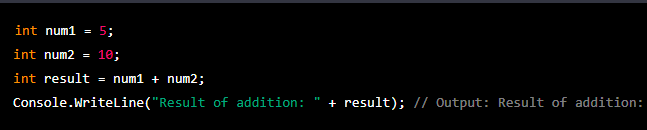2-Subtraction Operator (-):
Used to subtract two values.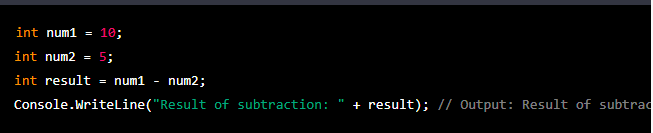3-Multiplication Operator (*):
Used to multiply two values.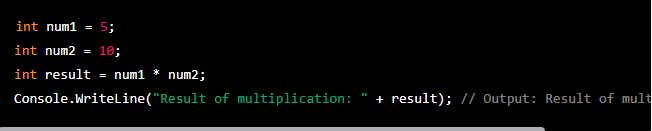4-Division Operator (/):
Used to divide two values.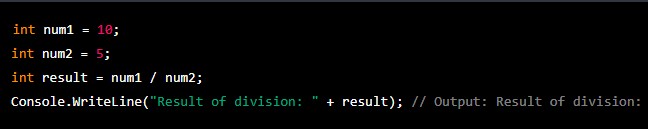Note that if you divide two integers, the result will also be an integer. If you want to get a decimal result, you should use a float or double data type.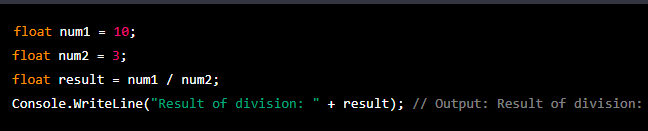5-Modulus Operator (%):
Used to return the remainder of a division operation.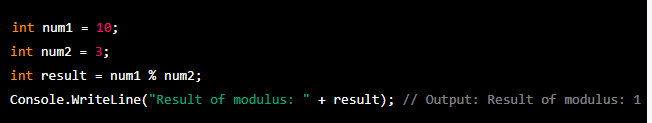In this example, the result of the modulus operation is 1 because 10 divided by 3 leaves a remainder of 1.

Comparison operators:
In C# are used to compare two values and return a Boolean value (true or false) depending on whether the comparison is true or false. There are six comparison operators in C#:
1-Equality Operator (==):
Used to check whether two values are equal.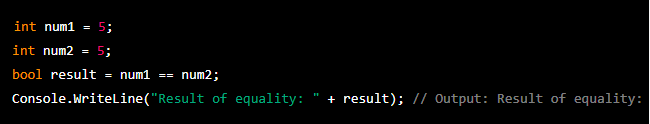2-Inequality Operator (!=):
Used to check whether two values are not equal.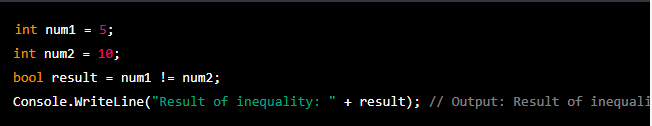3-Greater Than Operator (>):
Used to check whether the first value is greater than the second value.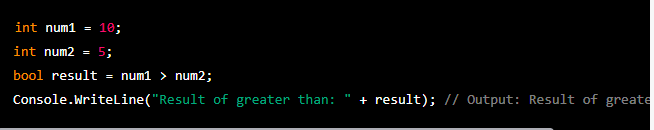4-Less Than Operator (<):
Used to check whether the first value is less than the second value.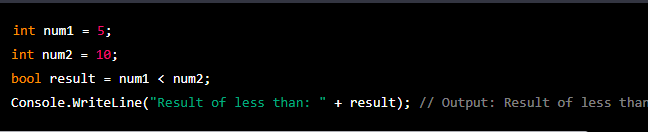5-Greater Than or Equal To Operator (>=):
Used to check whether the first value is greater than or equal to the second value.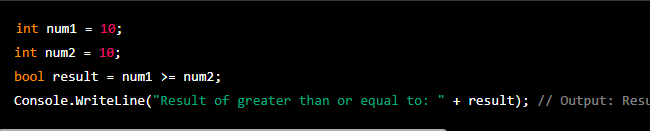6-Less Than or Equal To Operator (<=):
Used to check whether the first value is less than or equal to the second value.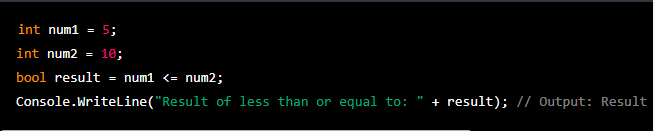In each of the above examples, the result variable will be assigned a Boolean value of true or false depending on whether the comparison is true or false.
Logical operators :
In C# are used to combine Boolean expressions and evaluate the result as either true or false. There are three logical operators in C#:
1-Logical AND (&&):
Returns true if both operands are true, otherwise returns false.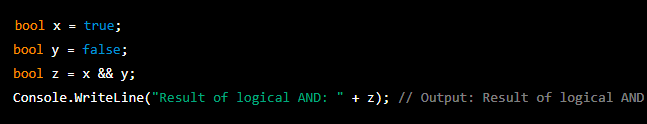In this example, the value of z will be false because x is true and y is false.
2-Logical OR (||):
Returns true if either operand is true, otherwise returns false.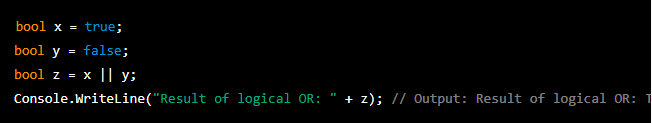In this example, the value of z will be true because x is true, even though y is false.
3-Logical NOT (!):
Returns true if the operand is false, and vice versa.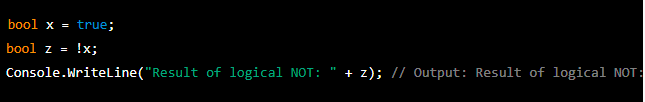In this example, the value of z will be false because the ! operator reverses the value of x, which is true.

Logical operators are often used in control flow statements such as if-else statements, while loops, and for loops to evaluate conditions.
For example: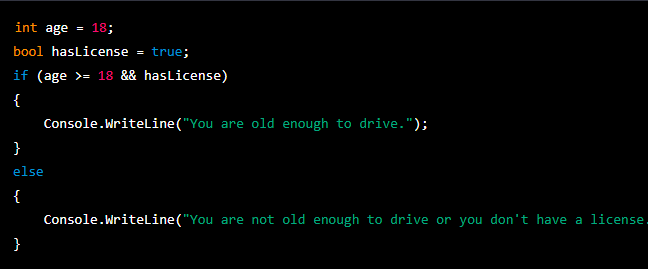In this example, the if statement checks whether the age variable is greater than or equal to 18, and whether hasLicense is true, using the logical AND operator (&&). If both conditions are true, the message "You are old enough to drive." will be printed. If either condition is false, the message "You are not old enough to drive or you don't have a license." will be printed.
Assignment operators:
used to assign values to variables.
Example: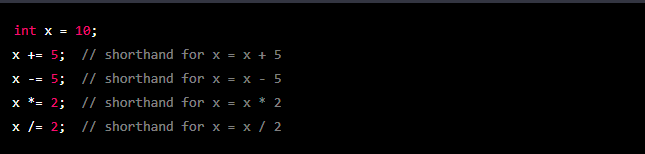Bitwise operators :
Bitwise operators are used to perform bitwise operations on binary numbers. These operators work on the individual bits of a number, rather than the entire number itself. Here are the bitwise operators in C#:

1-& (Bitwise AND): Returns 1 if both bits are 1, otherwise returns 0.
2-| (Bitwise OR): Returns 1 if either bit is 1, otherwise returns 0.
3-^ (Bitwise XOR): Returns 1 if exactly one bit is 1, otherwise returns 0.
4-~ (Bitwise NOT): Inverts all bits in a number.
5-<< (Left Shift): Shifts the bits of a number to the left by a specified number of positions.
6->> (Right Shift): Shifts the bits of a number to the right by a specified number of positions.
Here are some examples of using bitwise operators in C#: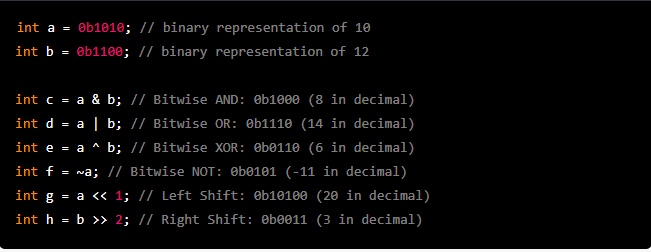In the first example, the & operator performs a bitwise AND operation on the binary representations of a and b, resulting in the binary number 0b1000, which is equal to 8 in decimal.
In the second example, the | operator performs a bitwise OR operation on the binary representations of a and b, resulting in the binary number 0b1110, which is equal to 14 in decimal.
In the third example, the ^ operator performs a bitwise XOR operation on the binary representations of a and b, resulting in the binary number 0b0110, which is equal to 6 in decimal.
In the fourth example, the ~ operator performs a bitwise NOT operation on the binary representation of a, resulting in the binary number 0b0101, which is equal to -11 in decimal due to two's complement representation.
In the fifth example, the << operator performs a left shift on the binary representation of a, shifting all the bits to the left by one position and inserting a 0 at the least significant bit. The resulting binary number 0b10100 is equal to 20 in decimal.
In the sixth example, the >> operator performs a right shift on the binary representation of b, shifting all the bits to the right by two positions and discarding the least significant bits. The resulting binary number 0b0011 is equal to 3 in decimal.

Common operator in C-Sharp in Hindi:
Common operators C# me mathematical, relational aur logical operations perform karne ke liye use kiye jaate hai. Kuch common operators niche diye gaye hai:
1-Arithmetic Operators:
In operators se hum mathematical operations jaise addition, subtraction, multiplication, division, modulus, etc. perform kar sakte hai.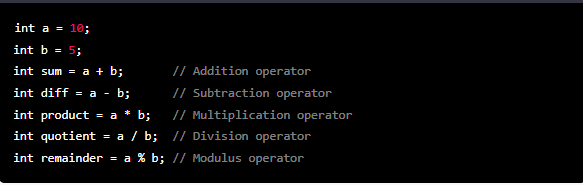2-Relational Operators:
Relational operators se hum values ko compare kar sakte hai, aur result true ya false return hota hai.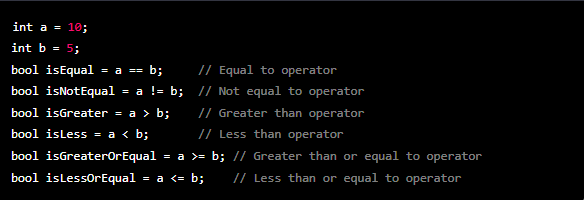3-Logical Operators:
Logical operators se hum multiple conditions ko check kar sakte hai, aur result true ya false return hota hai.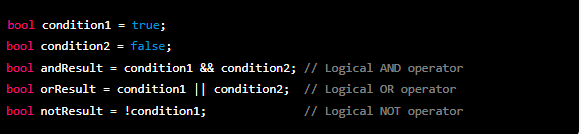4-Assignment Operators:
Assignment operators se hum variables me values assign kar sakte hai.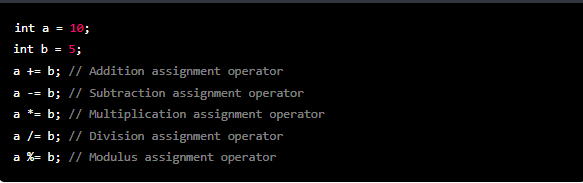5-Bitwise Operators:
Bitwise operators se hum bit level par operations perform kar sakte hai.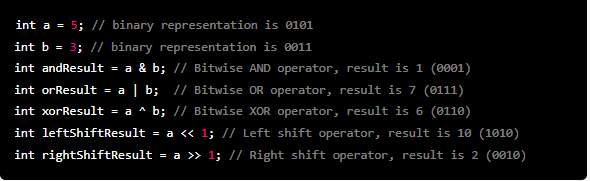Is tarah se, common operators ka use karke hum apne program ko functional aur dynamic bana sakte hai.

Thank You#### Brijesh Kumar

Database Developer

I have more then 6 years Experience in Microsoft Technologies - SQL Server Database, ETL Azure Cloud - Azure SQL Database, CosmosDB, Azure Data Factory, PowerBI, Web Job, Azure Function, Azure Storage, Web Apps, Powershall and Database Migration On-Premise to Azure Cloud.